Courses
Courses for Kids
Free study material
Free LIVE classes
More

# Curved SurfaceLIVE
Join Vedantu’s FREE Mastercalss

## Introduction to Curved Surface

You might have various closed objects like your lunch box, water bottle, a rolled wire, and so on. What are they actually? Well, they are shapes. Now, if I ask you what you see in the image below, please mark your answer: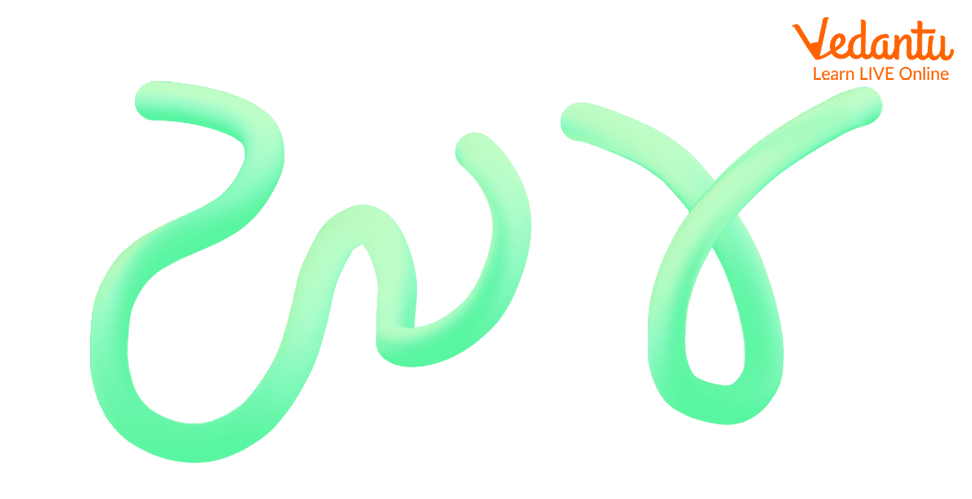Well, these are simply wires that are rolled in different forms. However, in Maths, we call these randomly folded wires curves. So, what are curves basically? Well, a curve is an abstract term used to describe the path of a continuously moving point. We notice that these curves are made of a straight line bent to form different shapes called curves.

Now, let us understand various types of curves and also the difference in each.

## Definition of a Curve

A curve is a smoothly flowing continuous line that has bent. It does not have any sharp turns. The technique to identify the curve is that the line bends and changes its direction at least once for all.

Various curve shapes other than the ones mentioned in the above image are circles, ellipses, parabolas, and hyperbolas, even arcs, sectors, and segments, they are all two-dimensional curved shapes. However, curves are three-dimensional shapes as well, such as spheres, cylinders, and cones; we all have these three-dimensional curved shapes.

## Different Types of Curves

Curves are categorised into different forms. Let us have a look at different types of curves with their representations:

## Simple Curve

A curve that changes its direction and does not intersect itself is a simple curve. A simple curve may be open or closed. This is what it looks like: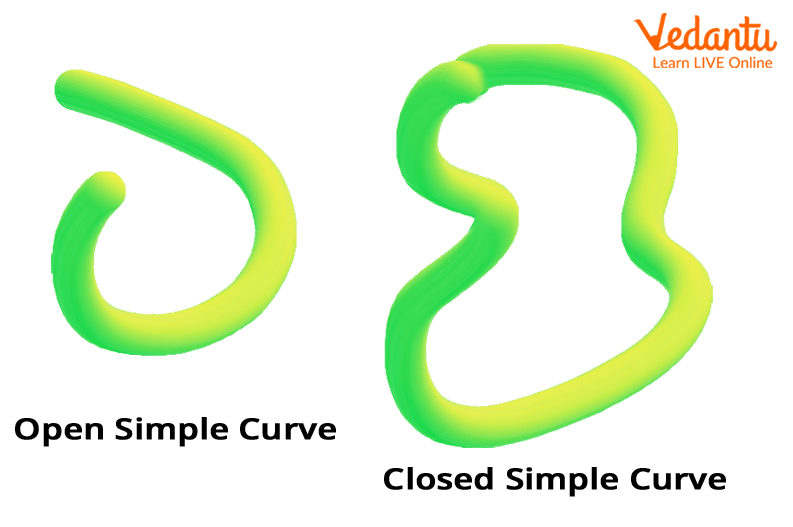## Non-Simple Curve

A type of curve other than a simple curve that crosses its path is a non-simple curve. It means the curve intersects itself while altering its direction. This is what it looks like: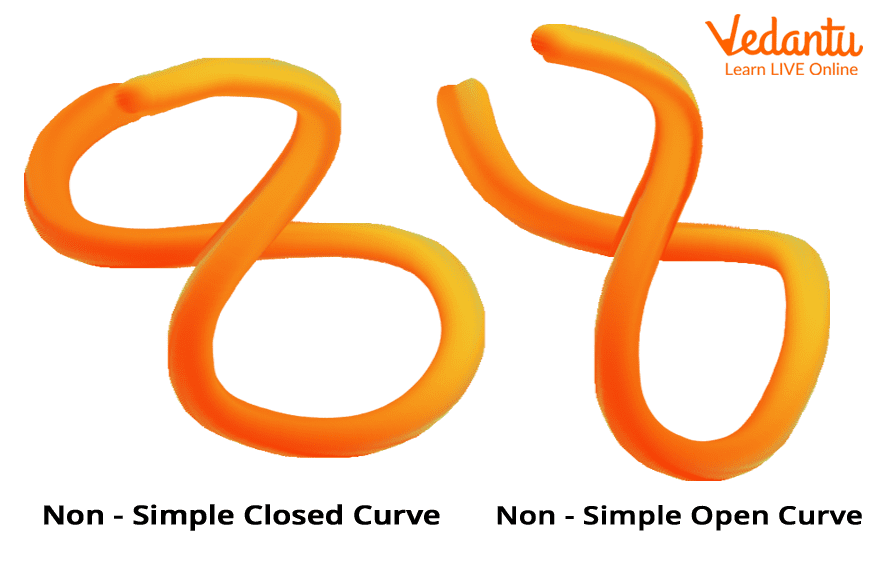## Open Curve

A curve that has two endpoints and does not enclose the area within itself is known as an open curve. Some of the examples of open curves are shown below: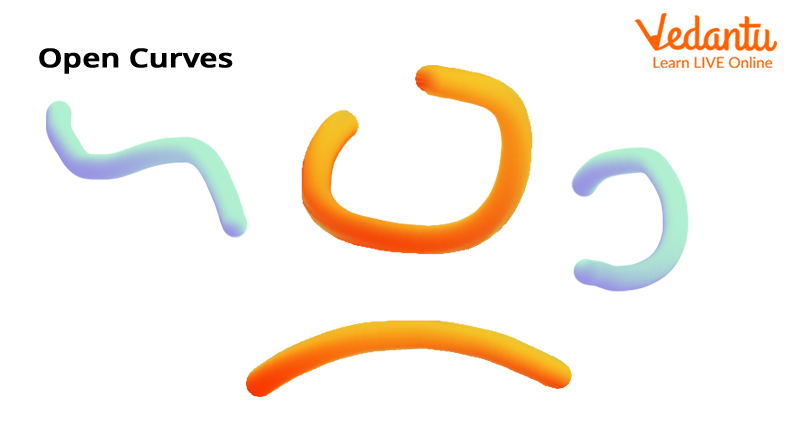## Closed Curve

A curve that has no endpoints and when it encloses the region an area forms, such a type of curve is known as the closed curve. A closed curve is formed by joining the two endpoints of the open curve. The best example of such types of curves are circles, ellipses, etc.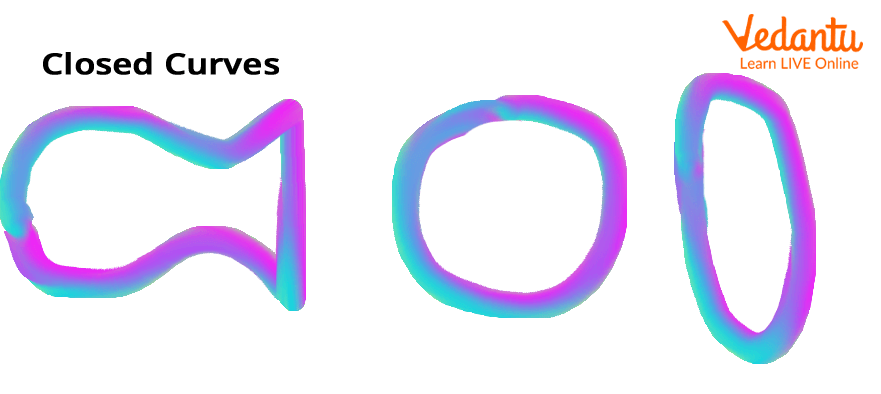## Curved Line

A bent line is called a curved line. If the curvature is not zero, we consider it a curve line, which is generally smooth and continuous.

• Curved Line Images

We observe many objects in our surroundings that are in the shape of a curved line. Some of them incorporate the following:

• Railways at the turning points,

• Track of a roller coaster,

• Paths of roads in hilly areas, and so on.

Apart from the real-life examples, we can also observe the curve-shaped lines in Maths; for example, the graph of a quadratic polynomial including parabola, ogive curve, arrows, etc.

So, this is how we understand curve Maths and the types of curves we find in our surroundings.

Last updated date: 28th Sep 2023
Total views: 141k
Views today: 3.41k

## FAQs on Curved Surface

1. What is a curve with an example?

A curve is a shape or a line that is evenly drawn on a surface having a bent or turned in it. For example, an ellipse and a circle are some examples of curved shapes.

2. What is the upward and downward curve?

• Upward Curve: An upward curve points towards the upward direction. The upward curves are called concave upward or convex downward curves.

An example of an upward curve is “U”.

• Downward Curve: A curve that points towards the downward direction is called a downward curve. These types of curves are also called concave downward or convex upward curves.

An example of a downward curve is “∩”.